# SAT Math Multiple Choice Question 199: Answer and Explanation

### Test Information

Question: 199

4. Newton's law of gravitation describes the strength of the force F between two objects with masses M and m separated by a distance of r units and is defined as. F =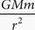. Which of the following gives the value of Newton's gravitational constant G, in terms of F, M, m, and r ?

• A. G = Fr2Mm
• B. G =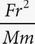• C. G =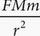• D. G =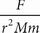B Plugging In could work on this one, but calculators aren't permitted. Since the equation is fairly simple, solving may be a better approach. Multiply both sides of the equation by r2 to get Fr2 = GMm. Divide both sides of the equation by Mm to get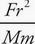= G. The correct answer is (B).